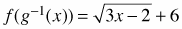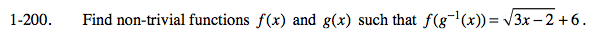### Home > CALC > Chapter 1 > Lesson 1.5.1 > Problem1-200

1-200.

Find non-trivial functions f(x) and g(x) such that. Homework Help ✎Let g −1(x) = 3x − 2.

What is g(x)?

What is f(x)?